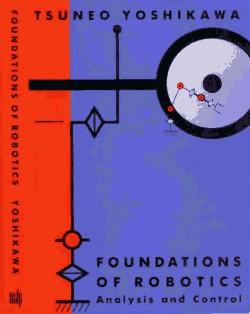# "Foundations of Robotics" (MIT Press, 1990)

by Tsuneo YOSHIKAWA

Some sample chapters are shown below.Sample chapters indicated in blue can be viewed.

```Preface

1  0verview of Robotic Mechanisms and Controller
l.l Mechanisms
1.2 Controller
Exercises
References

2  Kinematics
2.1 Position and Orientation of Objects
2.1.1 Object Coordinate Frame
2.1.2 Rotation Matrix
2.1.3 Euler Angles
2.1.4 Roll, Pitch, and Yaw Angles
2.2 Coordinate Transformation
2.2.1 Homogeneous Transform
2.2.2 Product and Inverse of Homogeneous Transform
2.3 Joint Variables and Position of End Effector
2.3.1 General Relation
2.3.4 Solution to Direct Kinematics Problem
2.4 Inverse Kinematics Problem
2.5 Jacobian Matrix
2.5.1 Translational and Rotational Velocity of Objects
2.5.2 Definition of the Jacobian Matrix
2.5.3 Link Velocities of a Manipulator
2.5.4 General Expression of the Jacobian Matrix Jv
2.5.5 Joint Velocity for Achieving Desired End-Effector
Velocity
2.5.6 Singular Configurations
2.6 Statics and Jacobian Matrices
2.6.1 Equivalent Forces Represented in Different Frames
2.6.2 Joint Driving Force Equivalent to Force Applied to
Tip of Manipulator
Exercises
References

3  Dynamics
3.1 Lagrangian and Newton-Euler Formulations
3.2 Some Basics of Kinematics
3.2.1 Newton's Equation and Euler's Equation
3.2.2 Lagrange's Equation
3.3 Derivation of Dynamics Equations Based on Lagrangian
Formulation
3.4 Derivation of Dynamic Equations Based on Newton-Euler
Formulation
3.4.1 Basic Procedure of Newton-Euler Formulation
3.4.2 Link Accelerations of a Manipulator
3.5 Use of Dynamics Equations and Computational Load
3.5.1 Real-Time Control---Inverse Dynamics Problem
3.5.2 Simulation---Direct Dynamics Problem
3.6 Identification of Manipulator Dynamics
3.6.1 Identification Problem of Manipulators
3.6.2 Identiiication Scheme Based on Lagrangian
Formulation
Exercises
References

4  Manipulability
4.1 Manipulability Ellipsoid and Manipulability Measure
4.2 Best Configurations of Robotic Mechanisms from
Manipulability Viewpoint
4.2.2 SCARA-Type Robot Manipulator
4.2.3 PUMA-Type Robot Manipulator
4.2.4 0rthogona1-, Cylindrical-, and Polar-Coordinate
Manipulators
4.2.5 Four-Joint Robotic Finger
4.3 Various Indices of Manipulability
4.4 Dynamic Manipulability
4.4.I Dynamic-Manipulability Ellipsoid and Dynamic-
Manipulability Measure
Exercises
References

5  Position Control
5.1 Generatiftg a Desired Trajectory
5.1.1 Joint-Variable Scheme
5.1.2 Scheme for Position Variables of End Effector
5.2 Linear Feedback Control
5.2.I Effectiveness of Linear Feedback Control
5.2.2 Stability of Proportional and Differential Feedback
Control
5.3 Two-Stage Control by Linearization and Servo
Compensation
5.3.I Basic Concept of Two-Stage Control
5.3.2 Structure of Control System
5.3.3 Parallel Processing Scheme
5.4 Design and Evaluation of Servo Compensation
5.4.1 Linear Servosystem Theory
5.4.2 Stability Margin and Sensitivity
5.5 Decoupling Control
5.5.1 Theory of Decoupling Control For Nonlinear
Systems
5.5.2 Application to Manipulators
5.5.3 Consideration of Actuator Dynamics
Exercises
References

6  Force Control             ,
6.I Impedance Control
6.1.1 Passive-Impedance Method
6.1.2 Active-Impedance Method---One-Degree-of-
Freedom Case
6.I.3 Active-Impedance Method---General Case
6.2 Hybrid Control
6.2.1 flybrid Control via Feedback Compensation
6.2.2 Dynamic Hybrid Control
Exercises
References

7  Control of Redundant Manipulators
7.1 Redundant Manipulators
7.2.2 Basic Equations
7.2.3 Second Subtask Given by Desired Trajectory
7.2.4 Second Subtask Given by Criterion Function
7.2.5 Formulation as Instantaneous Optimization
Problem
7.3 Application to Avoiding Obstacles and Singularitie's
7.3.1 Avoiding Obstacles
7.3.2 Avoiding Singularities
7.4 Computational Method far Desired Joint Velocity
Exercises
References

Appendix 1  Function atan2

Appendix 2  Pseudo-Inverses

Appendix 3  Singular-Value Decomposition

Appendix 4  Lyapunov Stability Theory

Solutions to Sclectcd Exercises

Index
```